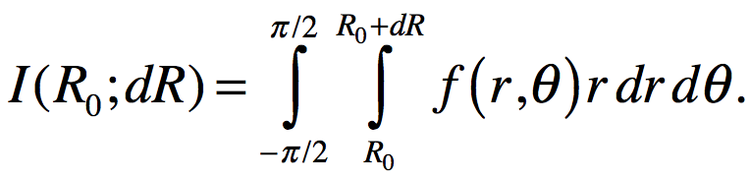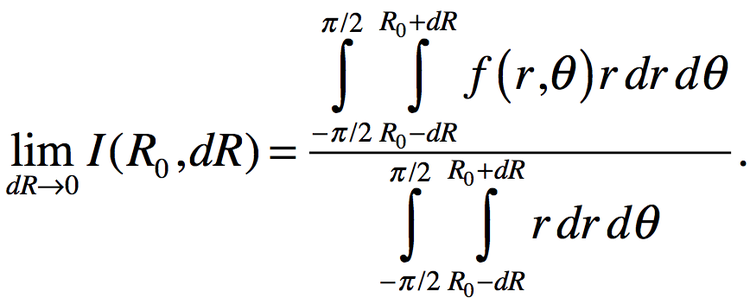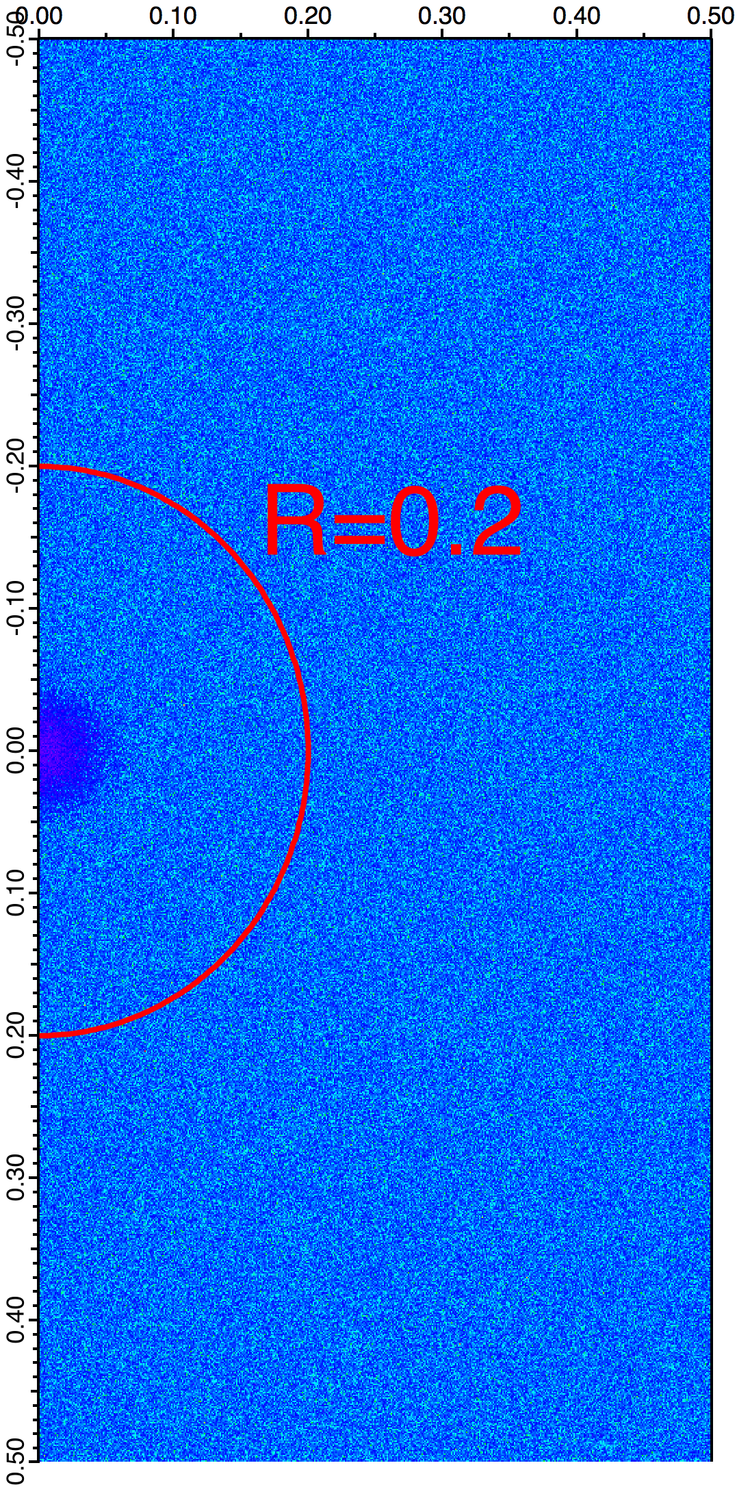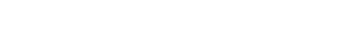# How to Average Data Over an Annular Domain

This blog post was inspired by a recent support question in which 2D data originated from an FFT of an image. The source of the data is important only to the extent that it adds a helpful symmetry condition. Here is an example:

Suppose you have an image of dimensions 1k x 1k:

`Make/O/N=(1000,1000) imageData=100*gauss(x,10,5,y,15,6)+enoise(0.05)  // sample`

We note that in practice the wave imageData may have application-dependent wave scaling but this is not immediately relevant.

You can compute the squared magnitude of the FFT using:

`FFT/OUT=4/DEST=xformData imageData`

The wave xformData is real (single precision floating point) with dimensions (501,1000).  Here the first dimension 501=1+(1000/2) is approximately half the size of the image because the Fourier transform of real valued data has the property that negative frequencies of the first dimension are generated by reflection about the Y-axis.

To estimate the average power spectral density we need to compute the integral of the data in an annular domain centered at the origin. In this case because of symmetry our domain is defined by radial distances R0 and R0+dR and angles theta=-pi/2 to pi/2. Mathematically the integral has the form:Here f(r,θ) represents our data in polar coordinates.

You can use the operation Integrate2D to compute the integral.  Before executing the operation you need to add the integrand function to your procedure window:

Function integrandFunc(pWave,inR,inTheta)
Wave/Z pWave
Variable inR,inTheta

Variable xx=inR*cos(inTheta)
Variable yy=inR*sin(inTheta)
Wave xformData=root:xformData       // point to the location of xformData
return inR*Interp2d(xformData,xx,yy)        // the integrand is r*f(r,theta)
End

Note that this integrand function uses polar coordinates as input parameters and only computes the Cartesian components for Interp2d(). Also note that xformData could be specified via pWave.

You can now compute the integral by executing the command:

`Integrate2D outerLowerLimit=R0, outerUpperLimit=(R0+dr), innerLowerLimit=(-pi/2), innerUpperlimit=(pi/2), integrand=integrandFunc`

If you are only be interested in the average value of the data at some radius R, you need to evaluateThe integral in the denominator can be expressed in closed form but it is easier to evaluate using Integrate2D with the following integrand function:

Function integrandFunc2(pWave,inR,inTheta)
Wave/Z pWave
Variable inR,inTheta

return inR
End

You can also use ImageLineProfile to compute the average value at radius R.  To do so you could start with a pair of waves that define the path over which you are averaging the data.  The following function creates the pair of waves that define the semi-circle of radius R_var:

Function makePath(R_var)
Variable R_var

Make/O/N=(181) xxx,yyy
Variable i,alpha,da
alpha=-90
for(i=0;i<=180;i+=1)
xxx[i]=R_var*cos(alpha*pi/180)
yyy[i]=R_var*sin(alpha*pi/180)
alpha+=1
endfor
EndFigure 1:  xformData with semicircle path of radius 0.2.

Using the path waves you can execute ImageLineProfile with width=0:

`ImageLineProfile xWave=xxx,yWave=yyy,width=0,srcWave=xformData`

and finally compute the average using:

`Print Sum(W_ImageLineProfile)/numpnts(W_ImageLineProfile)`

Note that if your path waves define a path that exits the domain of the wave xformData your line profile may contain NaN values.

## COMPARING THE ESTIMATES

Both the integration method and the lineProfile method required us to enter parameters that affected the accuracy of the calculation. The integration method requires that we specify dR while the line profile method requires that we choose the number of points in the path. In both cases we have limited accuracy because we are working with single precision data. To match estimates in this example we need dR ~ 0.00001 and approximately 2000 line profile points.ForumSupportGallery